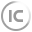NB-IoT的低功耗是怎么实现的

keil支持__at命令么

### 0

#include "pic.h"__CONFIG(FOSC_INTOSC&WDTE_OFF&MCLRE_ON); //配置字一，选择内部RC振荡器，关闭看门狗RA3作为复位引脚__CONFIG(BOREN_OFF&LVP_OFF&PLLEN_OFF);  //配置字二，关闭低电压复位，关闭低电压编程，关闭时钟4倍频//不同编译器版本的配置字写法可能不同，此为采用PICC 9.83版本的编译器配置字以上是人家写的程序配置位，我想请问大家BOREN_OFF、LVP_OFF、PLLEN_OFF这三个宏定义在哪里文件里，要是能找到我就能配置其他的位

### 6

51单片机学了也有半年了，各个模块也都了解，参考视频的教程也都可以理解。现在就是说不知道该怎么学了，是去网上找一找关于51单片机的实例去自己做一做，还是说在把51的内容加上数电和模电在好好的学一学啊。其实我自己希望是通过实践然后不断练习进步，但是现在好像没有什么方法，请求各位给我提出一些意见

### 5

#include<reg52.h>sbit a=P0^0;sbit b=P0^1;sbit c=P0^2;sbit OE0=P0^3;sbit OE1=P0^4;sbit OE2=P0^5;sbit KEY1=P3^2;sbit KEY2=P3^3;sbit KEY3=P3^4;sbit KEY4=P3^5;unsigned char code LedChar={{0xC30x810x000x000x000x810xFF0xFF}{0xFF0x810x000x000x000x810xFF0xFF}{0xFF0xFF0x000x000x000xFF0xFF0xFF}{0xFF0xFF0xFF0x000xFF0xFF0xFF0xFF}     //不按按键时{0xEF0xDF0xBF0x000xBF0xDF0xEF0xFF}     //左转{0xF70xFB0xFD0x000xFD0xFB0xF70xFF}      //右转{0xFF0xFF0xFF0xFF0xFF0xFF0x660x99}      //W };     unsigned char LedBuff={0xFF0xFF0xFF0xFF0xFF0xFF0xFF0xFF0xFF0xFF0xFF0xFF0xFF0xFF0xFF0xFF};void buan();          //不按按键函数void ting();           //刹车时函数void you();            //左转时函数void zuo();            //右转时函数void main(){TMOD=0x01;TH0=0xFC;TL0=0x67;EA=1;ET0=1;TR0=1;while(1){   if(KEY1==0)  {   zuo();  }  else if(KEY2==0)  {   you();  }  else  {  buan();  }}}/@@*中断函数*/void zhongduan() interrupt 1         //用于显示刷新{ static unsigned char i=0;TH0=0xFC;TL0=0x67;P1=0xFF;switch(i){  case 0:a=0;b=0;c=0;OE0=0;OE1=1;OE2=1;i++;P1=LedBuff;break;  case 1:a=0;b=0;c=1;OE0=0;OE1=1;OE2=1;i++;P1=LedBuff;break;  case 2:a=0;b=1;c=0;OE0=0;OE1=1;OE2=1;i++;P1=LedBuff;break;  case 3:a=0;b=1;c=1;OE0=0;OE1=1;OE2=1;i++;P1=LedBuff;break;  case 4:a=1;b=0;c=0;OE0=0;OE1=1;OE2=1;i++;P1=LedBuff;break;  case 5:a=1;b=0;c=1;OE0=0;OE1=1;OE2=1;i++;P1=LedBuff;break;  case 6:a=1;b=1;c=0;OE0=0;OE1=1;OE2=1;i++;P1=LedBuff;break;  case 7:a=1;b=1;c=1;OE0=0;OE1=1;OE2=1;i++;P1=LedBuff;break;  case 8:a=0;b=0;c=0;OE0=1;OE1=0;OE2=1;i++;P1=LedBuff;break;  case 9:a=0;b=0;c=1;OE0=1;OE1=0;OE2=1;i++;P1=LedBuff;break;  case 10:a=0;b=1;c=0;OE0=1;OE1=0;OE2=1;i++;P1=LedBuff;break;  case 11:a=0;b=1;c=1;OE0=1;OE1=0;OE2=1;i++;P1=LedBuff;break;  case 12:a=1;b=0;c=0;OE0=1;OE1=0;OE2=1;i++;P1=LedBuff;break;  case 13:a=1;b=0;c=1;OE0=1;OE1=0;OE2=1;i++;P1=LedBuff;break;  case 14:a=1;b=1;c=0;OE0=1;OE1=0;OE2=1;i++;P1=LedBuff;break;  case 15:a=1;b=1;c=1;OE0=1;OE1=0;OE2=1;i=0;P1=LedBuff;break;  default:break;}}/@@*左转*/void zuo(){LedBuff=LedChar;LedBuff=LedChar;LedBuff=LedChar;LedBuff=LedChar;LedBuff=LedChar;LedBuff=LedChar;LedBuff=LedChar;LedBuff=LedChar;LedBuff=LedChar;LedBuff=LedChar;LedBuff=LedChar;LedBuff=LedChar;LedBuff=LedChar;LedBuff=LedChar;LedBuff=LedChar;LedBuff=LedChar;}/@@*右转*/void you(){LedBuff=LedChar;LedBuff=LedChar;LedBuff=LedChar;LedBuff=LedChar;LedBuff=LedChar;LedBuff=LedChar;LedBuff=LedChar;LedBuff=LedChar;LedBuff=LedChar;LedBuff=LedChar;LedBuff=LedChar;LedBuff=LedChar;LedBuff=LedChar;LedBuff=LedChar;LedBuff=LedChar;LedBuff=LedChar;}/@@*不按按键*/void buan(){static unsigned char cnt=0;static unsigned int jisu=0;static unsigned char f=0;LedBuff=LedChar[cnt];LedBuff=LedChar[cnt];LedBuff=LedChar[cnt];LedBuff=LedChar[cnt];LedBuff=LedChar[cnt];LedBuff=LedChar[cnt];LedBuff=LedChar[cnt];LedBuff=LedChar[cnt];LedBuff=LedChar;LedBuff=LedChar;LedBuff=LedChar;LedBuff=LedChar;LedBuff=LedChar;LedBuff=LedChar;LedBuff=LedChar;LedBuff=LedChar;for(f=0;f>50;f++);jisu++;if(jisu>=400){  jisu=0;  cnt++;  if(cnt>=4)  {   cnt=0;  }}}芯片用的是74hc138

### 2

vl53l0x长距离模式怎么通过寄存器设置？

### 4

lm311比较器和普通的lm393这种比较器有什么区别，除了393是双比较器，311是单比较器，还有什么区别• 帮助人数
• 0
• 获得赞数
• 0
• 一周积分
• 0

< 1 >